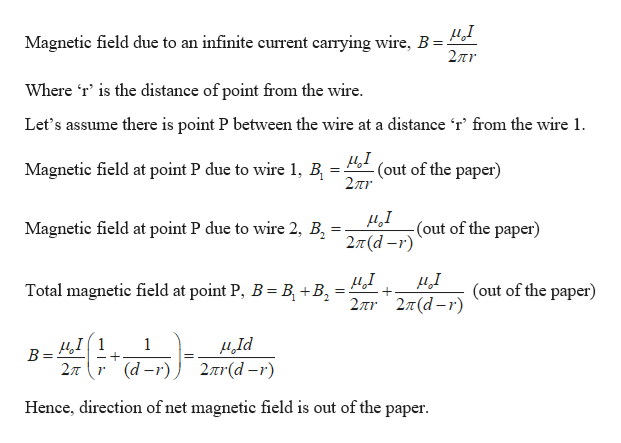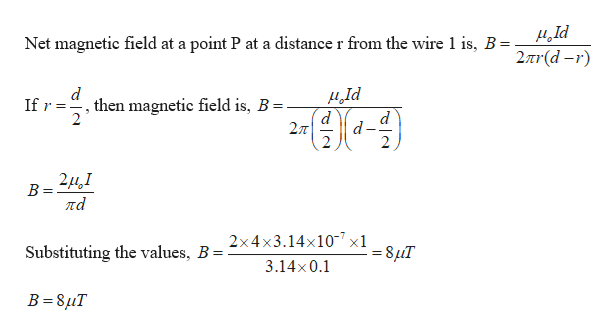# #2Pd#1

Question
4 views

Two very long wires are parallel to each other separated by a distance d.  The same current flows through each wire but in opposite directions.  See the arrows in the diagram.  Let I = 1 Amp and d = 0.1 meter.

a)  What is the direction of the net magnetic field due to the two, long wires in the space between the wires? Explain your answer.

b)  Calculate the magnitude of the net field at point P, which is half-way between the wires.

c)  Calculate the magnitude of the magnetic force per unit length due to wire #1 on #2.

d)  Determine the direction of the force calculated in part (c) and explain your answer.

check_circle

Step 1

Given:

Current in the wire, I = 1 amp

Length of the wire, d = 0.1 m

Step 2

(a) Calculating the direction of net magnetic field due to both wires in the space between them:help_outlineImage TranscriptioncloseMagnetic field due to an infinite current carrying wire, B 27r Where 'r' is the distance of point from the wire. Let's assume there is point P between the wire at a distance 'r' from the wire 1 (out of the paper) 2Tr Magnetic field at point P due to wire 1, B д,1 -(out of the paper) 2л (d -r) Magnetic field at point P due to wire 2, B Total magnetic field at point P, B B +B (out of the paper) 2πτ 2π(d - r) B=4I 2л Id 2r(d-r) 1 r (d-r) Hence, direction of net magnetic field is out of the paper fullscreen
Step 3

(b) Calculating the net magnetic field at ...help_outlineImage TranscriptioncloseNet magnetic field at a point P at a distance r from the wire 1 is, B = 2nr(d-r) Id d d 27T d If r,then magnetic field is, B = 2 2 В ла 2x4x3.14x107 x1 Substituting the values, B = -8uT 3.14x0.1 B 8uT fullscreen

### Want to see the full answer?

See Solution

#### Want to see this answer and more?

Solutions are written by subject experts who are available 24/7. Questions are typically answered within 1 hour.*

See Solution
*Response times may vary by subject and question.
Tagged in

### Current Electricity